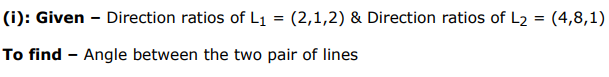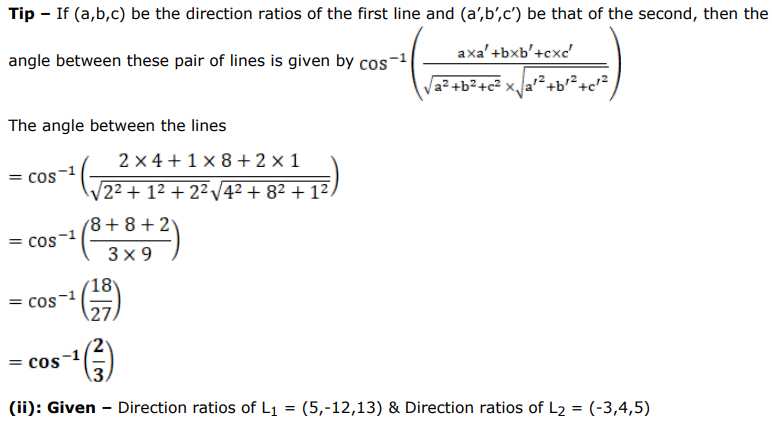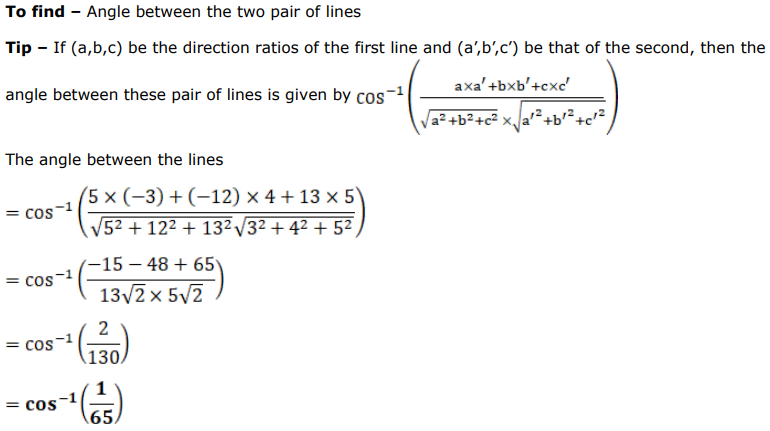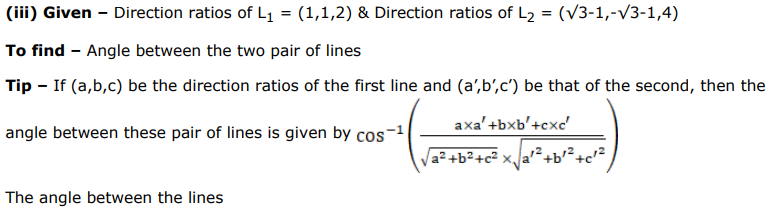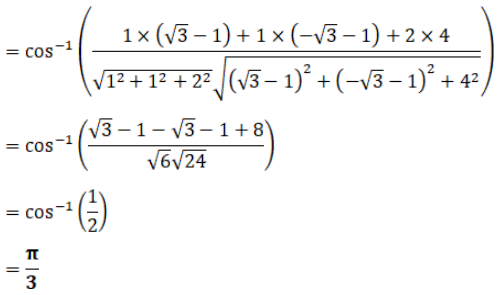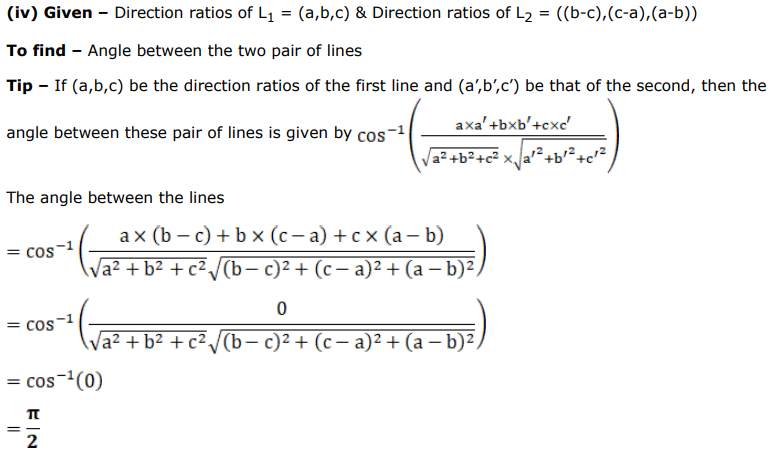# Find the angle between two lines whose direction ratios are

Question:

Find the angle between two lines whose direction ratios are

i. $2,1,2$ and $4,8,1$

ii. $5,-12,13$ and $-3,4,5$

iii. $1,1,2$ and $(\sqrt{3}-1),(-\sqrt{3}-1), 4$

iv. $a, b, c$ and $(b-c),(c-a),(a-b)$

Solution: